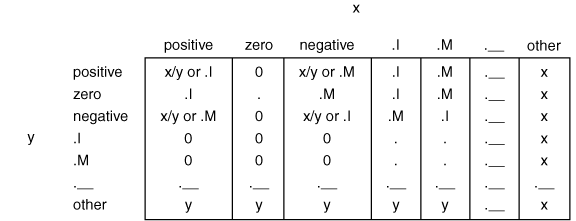Functions and CALL Routines

# DIVIDE Function

Returns the result of a division that handles special missing values for ODS output.
 Category: Arithmetic

## Syntax

 DIVIDE(x, y)

### Arguments

x

is a numeric constant, variable, or expression.

y

is a numeric constant, variable, or expression.

The DIVIDE function divides two numbers and returns a result that is compatible with ODS conventions. The function handles special missing values for ODS output. The following list shows how certain special missing values are interpreted in ODS:

• .I as infinity

• .M as minus infinity

• ._ as a blank

The following table shows the values that are returned by the DIVIDE function, based on the values of x and y.

Values That Are Returned by the DIVIDE FunctionNote:   The DIVIDE function never writes a note to the SAS log regarding missing values, division by zero, or overflow.The following example shows the results of using the DIVIDE function.

```data _null_;
a = divide(1, 0);
put +3 a= '(infinity)';
b = divide(2, .I);
put +3 b=;
c = divide(.I, -1);
put +3 c= '(minus infinity)';
d = divide(constant('big'), constant('small'));
put +3 d= '(infinity because of overflow)';
run;```

SAS writes the following output to the log:

```   a=I (infinity)
b=0
c=M (minus infinity)
d=I (infinity because of overflow)```Previous Page | Next Page | Top of Page